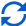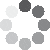Enter parameters Rise & Run
Enter parameters
Circular area
Rise:
in
• inches (in)
• feet (ft)
• yards (yd)
• centimeters (cm)
• meters (m)
Run:
in
• inches (in)
• feet (ft)
• yards (yd)
• centimeters (cm)
• meters (m)Result
Pitch:
-
Angle:
-
-
Rafter:
-
in
• inches (in)
• feet (ft)
• yards (yd)
• centimeters (cm)
• meters (m)
Enter parameters
Circular area
Rise:
in
• inches (in)
• feet (ft)
• yards (yd)
• centimeters (cm)
• meters (m)
Rafter:
in
• inches (in)
• feet (ft)
• yards (yd)
• centimeters (cm)
• meters (m)Result
Pitch:
?
Angle:
30
30
Run:
200
in
• inches (in)
• feet (ft)
• yards (yd)
• centimeters (cm)
• meters (m)
Enter parameters
Circular area
Run:
in
• inches (in)
• feet (ft)
• yards (yd)
• centimeters (cm)
• meters (m)
Rafter:
in
• inches (in)
• feet (ft)
• yards (yd)
• centimeters (cm)
• meters (m)Result
Pitch:
?
Angle:
30
30
Rise:
200
in
• inches (in)
• feet (ft)
• yards (yd)
• centimeters (cm)
• meters (m)(7 votes, average: 4.29 out of 5)Loading...
Similar Calculators:
Real-time graphics. Make the calculations and see the changes.
Real-time graphics. Make the calculations and see the changes.
Real-time graphics. Make the calculations and see the changes.
Embed
Similar Calculators:Contents:

## What is the roof pitch?

The roof pitch is the slope of the rafter.  The pitch is commonly defined as the ratio of rise over run in the form of x/12.

The rise is the height of the roof, and the run is the horizontal span (as pictured above).

For example, if a roof has a pitch of 4/12, then for every 12 inches the building extends horizontally, it rises 4 inches.

## How is roof pitch calculated with RISE and RUN?

It is a simple calculation using the formula:

$$Pitch = {Rise \over Run}$$

You’re probably wondering:

How can we compute the RAFTER LENGTH, GRADE, and ANGLE?

Once you know the rise and run, equations for a right triangle can be used to determine the rafter length, roof angle, and grade.

$$\text{Rafter length^2 = rise^2 + run^2}$$

This is the famous Pythagorean Theorem!

$$\text{tan (angle)} = {rise \over run}$$

and so

$$Angle = arctan{rise \over run}$$

The grade is the pitch expressed as a percentage:

$$Grade = \Bigr({rise \over run}\Bigr)100$$

And not only that:

The roof slope calculator also works if you only know the RISE and RAFTER (but not the RUN), or if you know the RUN and RAFTER (but not the RISE).

## How is roof pitch calculated with RISE and RAFTER or RUN and RAFTER?

If you know the RISE and RAFTER, the Pythagorean Theorem can be rearranged to obtain the RUN:

$$Run^2=rafret^2-rise^2$$

Similarly, if you know the RUN and RAFTER, the RISE is given by

$$Rise^2=rafter^2-run^2$$

Once you have both the RISE and RUN, the same equations as above can be used to obtain the remaining quantities.

## Step-by-step example

For this example, suppose you know the RISE and RAFTER lengths in inches.

1. Estimate the RISE and RAFTER lengths. Let’s assume they are 50 inches and 150 inches, respectively.
2. Use the Pythagorean theorem to calculate the RUN:
$$Run^2=rafter^2-rise^2= 150^2-50^2=20000$$
Taking the square root of both sides gives
$$Run = \sqrt{20000}=141.42 \;inches$$
3. Calculate the PITCH using
$${Rise \over Run} = {50 \over 141.42} = 0.354$$
We want the PITCH in the form x/12.  To get x, compute
$$x=12×{RISE \over RUN}=12×.354=4.2$$
Therefore, the pitch of your roof is 4.2/12.
4. You can also calculate the pitch ANGLE:
$$Angle = arctan(pitch) = arctan(0.354)= 19.5°$$
5. And lastly, calculate the GRADE:
$$Grade = 100×pitch= 100×.354 = 35.4%$$
Embed this calculator on your site!Add live graphics
Copied to clipboard! PreviewWhat's wrong?
Minimum 5 characters!